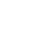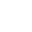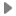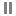# Graph – Find Cycle in Undirected Graph using Disjoint Set (Union-Find)

Objective: Given a graph, check if the graph contains a cycle using disjoint set.

Note: Disjoint-set data structure, also called a union–find data structure or merge–find set.

Example:Earlier in Detect Cycle in Undirected Graph using DFS we discussed about how to find cycle in graph using DFS. In this article we will discuss how to find cycle using disjoint-set.

How to find cycle:

• The makeset operation makes a new set by creating a new element with a parent pointer to itself.
• Then process each edge of the graph and perform find and Union operations to make subsets using both vertices of the edge.
• If find operation on both the vertices returns the same parent (means both vertices belongs to the same subset) then cycle is detected.

Lets walk through one example for more understanding, see the animation below:Java Code:

Output:

```Graph contains cycle: true
```

Ref: wiki

__________________________________________________
Top Companies Interview Questions..-

If you find anything incorrect or you feel that there is any better approach to solve the above problem, please write comment.
__________________________________________________